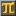#SCIENTIFIC CALCULATOR

This calculation: Edit - Share - Print -
Save

• Press Ctrl-d to save this calculation as a bookmark.

- Reset

 Approximate optimal body weight for adults Devine's formula Body height in centimeters: 180 [cm] Approx. optimal weight for men (kilograms): int((50 + 2.3 * ([cm] - 152.4) / 2.54) * 10) / 10 =   74.9  Approx. optimal weight for women: int((45.5 + 2.3 * ([cm] - 152.4) / 2.54) * 10) / 10 =   70.4  Hit the Edit link to adjust input for your height.
CalculatorPi.comⒸ 2012-2022 Troymius LLC